# Step hyperbolic cross

A summation domain of multiple Fourier series. Like a hyperbolic cross, it is used for good approximation in the space of functions with bounded mixed derivative (in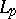).

Letbe an integrable periodic function ofvariables defined on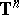. It has a Fourier series expansion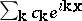,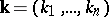,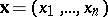,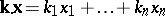. Unlike in the one-dimensional case, there is no natural ordering of the Fourier coefficients, so the choice of the order of summation is of great importance.

Let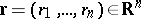with all coordinates positive,. Let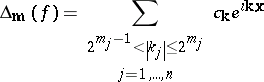be a dyadic "block" of the Fourier series. The step hyperbolic partial sums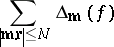where introduced by B. Mityagin [a2] for problems in approximation theory. They have approximately the same number of harmonics as a hyperbolic cross, but structurally they fit the Marcinkiewicz multiplier theorem (cf. also Interpolation of operators). It implies that the operator of taking step hyperbolic partial Fourier sums is bounded in each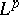,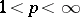. This means that step hyperbolic partial sums give the best approximation among all hyperbolic cross trigonometric polynomials in,. In the limit cases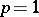and, the Lebesgue constants of step hyperbolic partial sums have only logarithmic growth, while for hyperbolic partial Fourier sums they grow as a power of.